# Frame

(diff) ← Older revision | Latest revision (diff) | Newer revision → (diff)

A set of linearly independent vectors taken in a definite order and placed at a common origin. Any three non-parallel vectors not lying in one plane can serve as a frame for the vectors in space. If the vectors building the frame are mutually orthogonal, then the frame is called orthogonal; if in this case the length of the vectors is equal to one, the frame is called orthonormal.

A framing of an-dimensional differentiable manifoldis a vector bundle isomorphism of its tangent bundle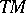with the trivial bundle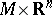(so thatis parallelizable). Using the standard basisofsuch an isomorphism defines a frame field: it assigns to everya frame, or basis, of the tangent space at that point.
The frame bundle over a manifoldis the principal fibre bundle with structure group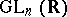whose fibre over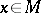is the collection of all bases (frames) of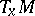, the tangent space at that point.
A-frame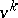inis an ordered set oflinearly independent vectors. Let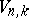denote the set of all-frames in. Let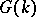be the subgroup ofleaving a fixed frame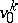invariant. Then. Thus,has a real-analytic structure. It is called the Stiefel manifold of-frames in-space.